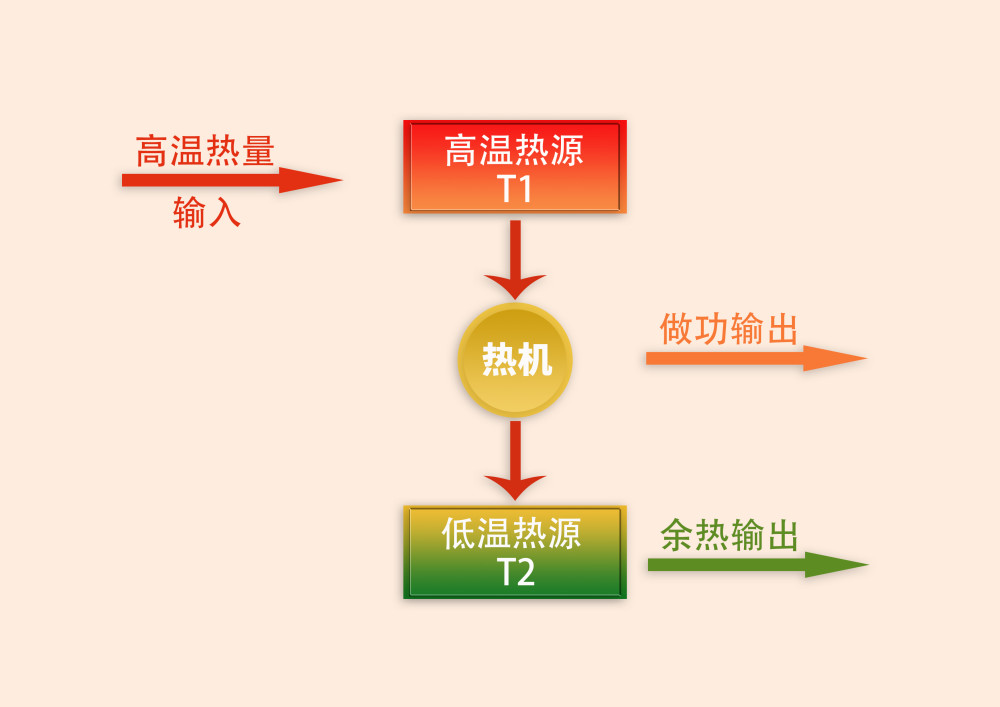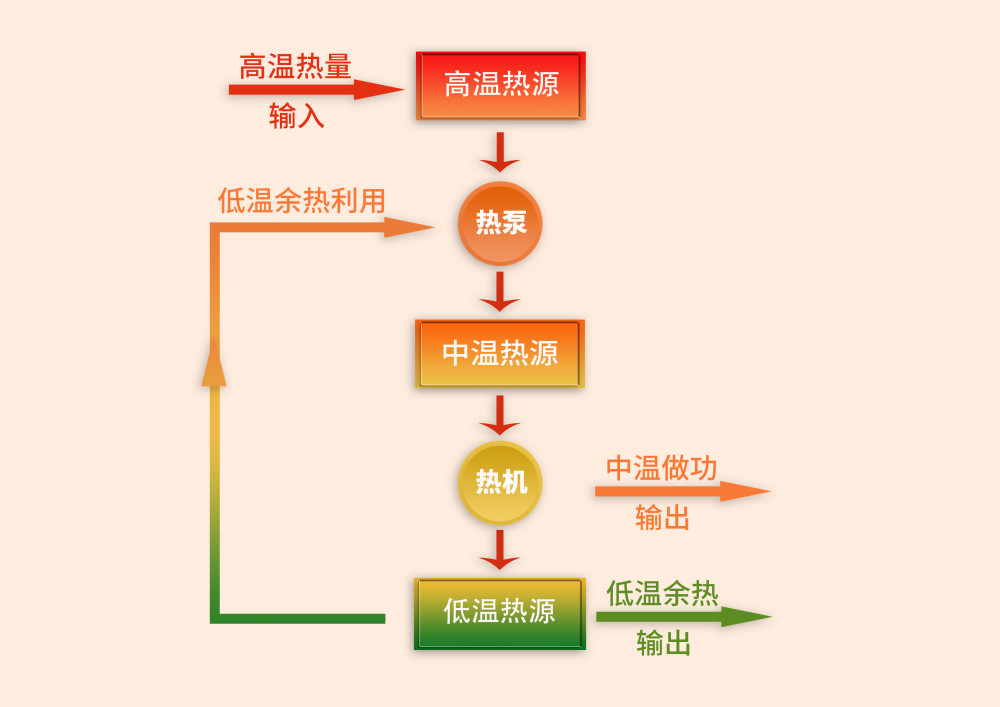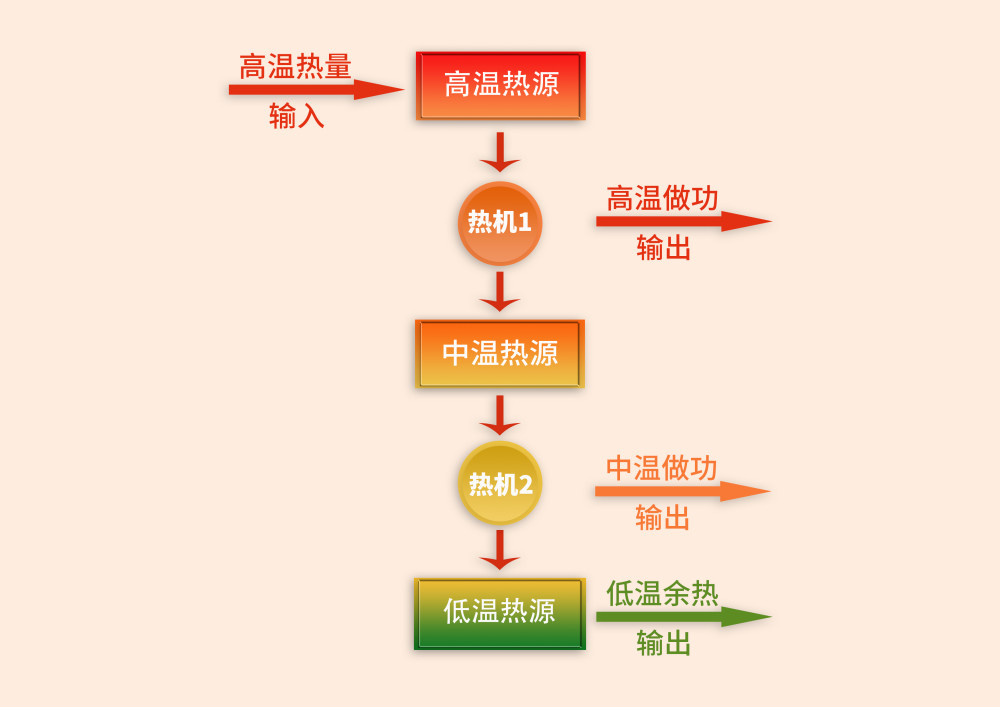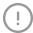360搜索与该网页作者无关，不对其内容负责。

# 从卡诺循环再认识谈理论的应用创新图一

1、低温热源温度难以降低?

2、温度越高效率越高?

3、过程热-功转化效率等于系统热-功效率?图二

Q0=Q1=W1+Q6

Q2=Q1+Q5

Q3=Q2=W1+Q4

Q4=Q5+Q6

Q2=Q1+Q5=4Q0

W1=25%Q2=Q0

Q4=Q2-W1=3Q0

Q6=Q4-Q5=0图三

《国际歌》有一句歌词唱得好:“要冲破思想的牢笼”。而一旦冲破思想的牢笼，走出思维定势，甩掉那根 “木桩”，我们的潜力将会得到极大释放，将会创造各种奇迹。举报